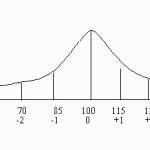# Normal Distribution Probability in Excel: All Versions up to 2016

Share on

Microsoft Excel for statistics > Normal Distribution Probability in Excel

Before you begin, you might find it helpful to review what a normal distribution is. See: What is a normal distribution?
You can also review what different types of word problems you’re likely to come across. See:
Normal distributions Word Problems.

Watch the video for the steps. Update 6/4/2016: Although I’m using an older version of Excel in this video, the steps are exactly the same for Excel 2016:

## How to Calculate Normal Distribution Probability in ExcelWhile it’s possible to look up probabilities for a normal distribution using the z-table, it’s actually much easier to calculate probabilities in Excel for a couple of reasons. First, there’s no looking at a table; the NORMDIST function does the hard work for you. Second, Excel does the intermediate calculations for you. Most calculation errors happen in an intermediate step (such as calculating the z-score to look up) rather than the actual z-score itself. Excel can handle three types of probability calculations: more than, less than, and in between. These instructions work for Excel 2007 and Excel 2010.

Sample Question: Suppose that a contaminant in samples from a city’s water supply has a mean of 500 ppm and a standard deviation of 100 ppm. What is the probability that bacteria in a randomly selected water sample will be: 1/ less than 600 ppm 2/More than 600 ppm 3/between 400 and 600 ppm?

### Calculate Normal Distribution Probability in Excel: Less than

Step 1: Click an empty cell.

Step 2: Click “Insert Formula”.

Step 3: Type “Normdist” into the search box and then click “Go.”

Step 4: Select “NORMDIST” from the list and then click “OK” to open the Function Arguments window.

Step 5: Enter your data into the box. For this example, type “600” in the X box, “500” in the Mean box, “100” in the Standard Deviation box and “true” in the cumulative box..

Step 6: Click “OK.”. This returns 0.84134474 in the cell you clicked in Step 1, which is the probability of getting under 600 ppm.

### Calculate Normal Distribution Probability in Excel: More than

1-0.84134474= 0.158653.

### Calculate Normal Distribution Probability in Excel: Between

Step 8: Repeat Steps 1 through 8 for the second value, which for this example is 400. The probability is 0.15865526.
Step 9: Subtract the larger probability from the smaller probability. In this example:
0.84134474 – 0.15865526 = 0.68269

That’s it!

## References

Agresti A. (1990) Categorical Data Analysis. John Wiley and Sons, New York.
Gonick, L. (1993). The Cartoon Guide to Statistics. HarperPerennial.
Vogt, W.P. (2005). Dictionary of Statistics & Methodology: A Nontechnical Guide for the Social Sciences. SAGE.
Wheelan, C. (2014). Naked Statistics. W. W. Norton & Company

CITE THIS AS:
Stephanie Glen. "Normal Distribution Probability in Excel: All Versions up to 2016" From StatisticsHowTo.com: Elementary Statistics for the rest of us! https://www.statisticshowto.com/how-to-calculate-normal-distribution-probability-in-excel/
---------------------------------------------------------------------------Need help with a homework or test question? With Chegg Study, you can get step-by-step solutions to your questions from an expert in the field. Your first 30 minutes with a Chegg tutor is free!